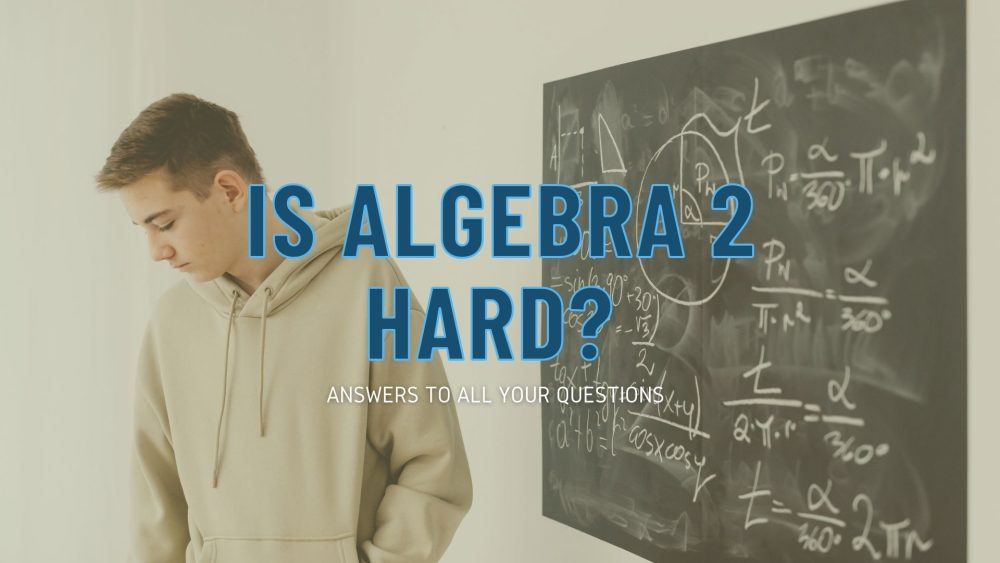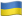# Is Algebra 2 Hard? Let’s Find Out!Is Algebra 2 hard? This is a question we get pretty often, so we’ve decided to write a comprehensive blog post about it. Algebra 2 is a relatively difficult class, but it doesn’t need to be. Truth be told, you shouldn’t have a major problem with this type of algebra if you have a firm grasp on the prerequisites: exponents, integer arithmetic, pre-algebra, fraction arithmetic, geometry, and Algebra 1.

However, keep in mind that there are many things hanging in the balance when it comes to Algebra 2. Regardless of their major, college students need to pass Algebra 2 to get their Bachelor’s degree. Some programs also require this for students looking to get an Associate’s Degree. If you are looking for science degrees, you will also need to pass Pre-calculus and Calculus. However, let’s focus on Algebra 2 for now.

## Is Algebra 2 Hard? Well, It Depends

Is algebra 2 easy? Why is algebra 2 so hard? These are two very important questions. Let’s take a look at some of the things that certainly make this course a difficult one for college students (or even high school students):

• The Algebra 2 course usually lasts for an entire year in high school, so it’s definitely a comprehensive course. Many colleges, however, have just a 6-month course. This is simply not enough to teach all students the algebraic manipulation skills and the arithmetic (exponents or fractions are two good examples) one needs to solve complex problems.
• Many professors tend to assume that their students already know some concepts introduced by Algebra 2. This makes solving hard algebra 2 equations very difficult for students.
• In many cases, students are not even proficient in Algebra 1, which also causes some problems.
• Things like finding the equation of the exponential or solving a quadratic equation with two or more complex solutions are pretty difficult to understand for most students. These exercises require a lot of time and effort to resolve. One also needs a lot of practice with these kinds of equations.

### Important Algebra 2 Concepts and Skills

Let’s talk about some Algebra 2 concepts and skills that students need to know before even considering passing the course. Basically, Algebra 2 will require you to master 12 major areas of mathematics:

1. Transformations of functions
2. Modeling
3. Complex numbers
4. Polynomial factorization
5. Equations
6. Rational exponents and radicals
7. Polynomial division
8. Polynomial graphs
9. Exponential models
10. Polynomial arithmetic
11. Trigonometry
12. Logarithms

Each area has a number of different things you should master. For instance, polynomial arithmetic requires you to know how to add or subtract polynomials, how to multiply polynomials by polynomials, and even obtain the average rate of change of polynomials.

As for the skills required to effectively solve Algebra 2 problems, you will need to master Algebra 1. And of course, the most important equation you need to learn how to solve is the classic “y=ax2 + bx + c”. Another very important skill you will need to master is the ability to apply the Algebra II concepts you learn to real-world problems.

Finally, many students ask us “do you need geometry for Algebra 2?” Even though Geometry is a prerequisite for Algebra 2 (so is Algebra 1), there isn’t really much to be scared about. You will encounter the occasional need for a geometry formula, most often in word problems. However, this does not mean you shouldn’t learn geometry. Remember, you need to pass that class if you want to get enrolled in Algebra 2 (and not prep track math class).

### The Difference Between Algebra 1 and 2

So, what’s the difference between Algebra 1 and 2? Most students who managed to understand Algebra 1 are wondering what’s in store for them during the Algebra 2 class. However, before we show you the differences between the two, we want to assure you that you can pass the course. You just need to dedicate more time to the Algebra 2 course. You need to practice. After all, practice makes perfect. You will also need to learn the ropes of mathematical writing. This also takes practice to master. Now, let’s take a look at some of the differences between the two courses:

 Algebra 1 Algebra 2 Overview You will learn the concepts behind algebra. This means learning numbers, functions, measurement, using rational expressions, exponents, inequalities, linear equations, coordinates, and so on. Algebra 2 is a more complex course that aims to teach you many other areas of mathematics. For instance, you will learn polynomials, logarithms and logarithmic expressions, polynomials, trigonometry, sequences and series, and so on. Level Algebra 1 is like an introduction to algebra, so you will have a basic understanding of the various concepts after you finish the course. Algebra 2 is more advanced and builds upon the things you’ve learned in Algebra 1 to help you understand difficult concepts. Life Application Algebra 1 is very important to master if you want to understand the concepts behind Algebra 2 problem solving. In Algebra 2, you will learn to apply the simple things you’ve learned in Algebra 1 to solve complex, real-life problems. What You Will Learn Equations and inequalities are two of the most important things you will learn in Algebra 1. Algebra 2 will teach you more advanced equations, like the logarithmic and exponential ones. You will also learn about polynomial calculus, as well as quadratic equations, matrices and graphs. Course Progression You can’t progress to the Algebra 2 course without passing the Algebra 1 class. You can’t be enrolled in the Calculus class without first passing the Algebra 2 class. Concepts Covered Algebra 1 works only with integers and rational numbers, which are not very difficult to understand and use. Algebra 2 introduces and discusses complex numbers and uses them to solve complex problems.

### How Hard Is Pre Calculus Compared to Algebra 2

How hard is Algebra 2? How hard is pre calculus compared to Algebra 2? These two questions are difficult to answer. As you can see from the information we have provided above, there is a big difference between Algebra 1 and 2. The latter is more difficult. However, how difficult it would be for you is not something we can predict. If you are relatively good at understanding mathematical concepts, you should do very well in Algebra 2.

Also, you shouldn’t be too worried about precalculus either. This is basically a more difficult version of Algebra 2 that aims to prepare you for the Calculus class. You will have to learn more trigonometry, as well as work with some pretty nasty decimals and fractions. You should also expect to do some complex factoring and division work. Other things students find difficult are reasoning and proofs, and sets and set operations. However, you shouldn’t worry too much about these if you are willing to dedicate the time and effort required by this class. And remember, if you run into any trouble, our expert writers (all are ENL writers, by the way) will help you solve any problem in no time.

### Solving Difficult Algebra 2 Problems Quickly

Unfortunately, there is no easy way to solve difficult Algebra 2 problems quickly. Otherwise, why would anyone consider math difficult? And no, there is no software that can solve any problem correctly. The best way to solve these problems quickly is to learn everything about basic algebraic concepts and operations, learn all the problem solving concepts used for quick solutions (like the principle of inverses), learn all the basic transformations, and perhaps learn a few useful patterns for solving specific equations.

### Learn How to Be Good at Algebra

Getting your professor to award you high grades in Algebra 2 is not as easy as you think. These professors usually have pretty high standards and expectations from their students. So you need to learn how to be good at algebra without spending all your weekends on it. Here are some of the best tips our experienced math problem solvers and technical writers can give you:

• Try to solve the problems that were discussed in class – after each and every class. This way, you will understand the solutions and will know how to apply them to solve more complex problems further down the line.
• If you don’t understand something, don’t be afraid to ask. Ask your classmates, your parents or even your professor. Also, you will find a lot of help (even guidelines on how to solve certain problems and equations) on the Internet.
• Get prep online. You will be amazed by how much you can learn when our math professional explains everything to you. Also, you can ask questions and get the perfect answers in minutes. What better way to learn math quickly?
• Study and then study some more. You will need a lot of practice to solve complex problems, so it is extremely important to commit the time and effort necessary to understand all the concepts. If you fall behind, you’ll find it very difficult to catch up.
• Practice solving problems. Don’t just real the theoretical framework. Make sure you work on real equations, trying to solve them.
• Organize your time and sleep well. Most students get desperate before a test and spend day and night trying to learn Algebra 2. This is not the way to go. You will just burn yourself out in record time. To be efficient, you need to organize your time and dedicate two or three hours every day to mathematics. You can take the weekend off.
• You should not rely on online calculators or equation-solving software. These will just give you the answer. Your professor wants to see how you solve the problem step by step. You won’t get a decent grade using math programs, guaranteed.

### Can Somebody Do My Math Homework?

I think I need somebody to do my math. Where could I get some affordable assistance from? College and university students sometimes need some math help. It’s not something to be ashamed about. Trust us, our math experts help hundreds of students every month. You can get top-notch assistance with your math homework from the best experts on the Internet in mere minutes right here. Also, we offer tutoring and prep services for people who want to excel at not only Algebra 2, but also precalculus and even calculus.

We have experts online day and night, so you can get the help you need as fast as possible. Speaking of our experts, did you know that they all have at least a PhD degree in a field in mathematics? You will get custom academic content from a team of genuine experts who know what they’re talking about. Many of our experts are also former teachers, so they know exactly what your professor wants to see. Get cheap help from our company and we’ll make learning Algebra 2 a piece of cake!

Let's stand with the heroesAs Putin continues killing civilians, bombing kindergartens, and threatening WWIII, Ukraine fights for the world's peaceful future.

Ukraine Live Updateshttps://war.ukraine.ua/
Donate Directly to Ukraine